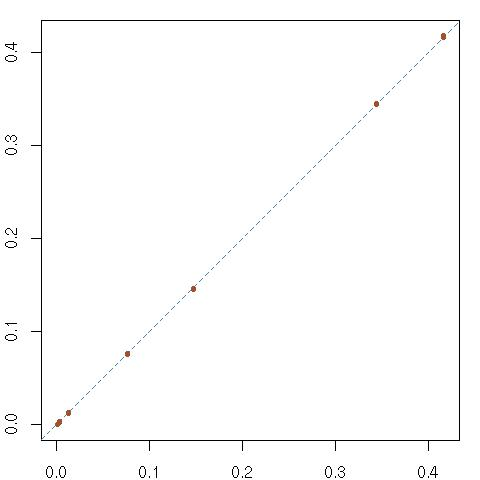# Random sudokus [p-values]

May 21, 2010
By

Want to share your content on R-bloggers? click here if you have a blog, or here if you don't.I reran the program checking the distribution of the digits over 9 “diagonals” (obtained by acceptable permutations of rows and column) and this test again results in mostly small p-values. Over a million iterations, and the nine (dependent) diagonals, four p-values were below 0.01, three were below 0.1, and two were above (0.21 and 0.42). So I conclude in a discrepancy between my (full) sudoku generator and the hypothesised distribution of the (number of different) digits over the diagonal. Assuming my generator is a faithful reproduction of the one used in the paper by Newton and DeSalvo, this discrepancy suggests that their distribution over the sudoku grids do not agree with this diagonal distribution, either because it is actually different from uniform or, more likely, because the uniform distribution I use over the (groups of three over the) diagonal is not compatible with a uniform distribution over all sudokus…

R-bloggers.com offers daily e-mail updates about R news and tutorials about learning R and many other topics. Click here if you're looking to post or find an R/data-science job.
Want to share your content on R-bloggers? click here if you have a blog, or here if you don't.

Tags: , , , , , , , , ,